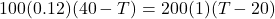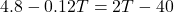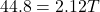## In the lab, you submerge 100 g of 40°C nails in 200 g of 20°C water. (The specific heat of iron is 0.12 cal/g # °C.) Equate the heat gained

Question

In the lab, you submerge 100 g of 40°C nails in 200 g of 20°C water. (The specific heat of iron is 0.12 cal/g # °C.) Equate the heat gained by the water to the heat lost by the nails, and show that the final temperature of the water is about 21°C.

in progress 0
2 months 2021-08-03T16:56:12+00:00 1 Answers 2 views 0

Final temperature of the mixture becomes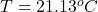Explanation:

Here we know that heat given by the nail is equal to the heat absorbed by water

So here we know that heat to change the temperature is given as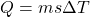so by equating the heat we have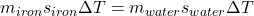now we have